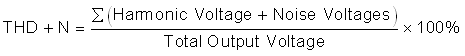SLOA011B January   2018  – July 2021

1. 1Introduction
2. 2Non-Inverting Amplifier
3. 3Inverting Amplifier
4. 4Simplified Op Amp Circuit Diagram
5. 5Op Amp Specifications
6. 6References
7. 7Glossary
8. 8Revision History

## 5.15 Total Harmonic Distortion Plus Noise

Total harmonic distortion plus noise, THD + N, compares the frequency content of the output signal to the frequency content of the input. Ideally, if the input signal is a pure sine wave, the output signal is a pure sine wave. Because of non-linearity and noise sources within the op amp, the output is never pure. THD + N is the ratio of all other frequency components to the fundamental and is usually specified as a percentage:

Equation 35.Figure 5-10 shows a hypothetical graph where THD + N = 1%. The fundamental is the same frequency as the input signal and makes up 99% of the output signal. Non-linear behavior of the op amp results in harmonics of the fundamental being produced in the output. The noise in the output is mainly due to the input referenced noise of the op amp. All the harmonics and noise added together make up 1% of the output signal.

Two major reasons for distortion in an op amp are the limit on output voltage swing and slew rate. Typically an op amp must be operated at or below its recommended operating conditions to realize low THD.Figure 5-10 Output Spectrum with THD + N = 1%